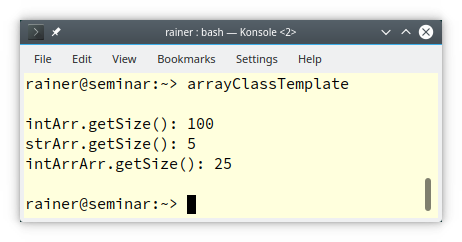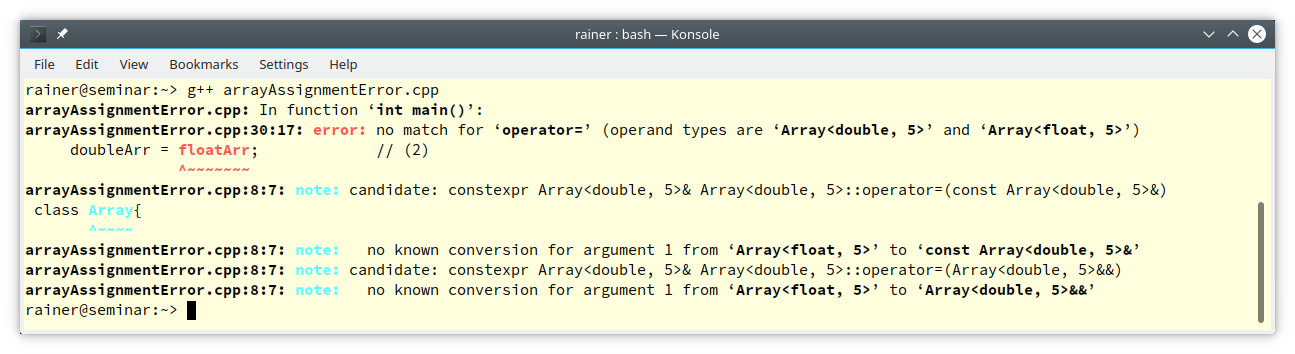# Class Templates

A function template represents a family of functions. Accordingly, a class template represents a family of classes. Today, I want to introduce class templates.Defining a class template is straightforward.

## Definition of a Class Template

Assume you have a class A`rray` that should become a class template.

```class Array{

public:
int getSize() const {
return 10;
}

private:
int elem;
};
```

##Modernes C++ Mentoring

Be part of my mentoring programs:

• "Fundamentals for C++ Professionals" (open)
• "Design Patterns and Architectural Patterns with C++" (open)
• "C++20: Get the Details" (reopens December 2023)
• Do you want to stay informed about my mentoring programs: Subscribe via E-Mail.

The class `Array` holds a C-array of int with length 10. The type of the C-array and its length are obvious generalization points. Let’s make a class template by introducing a type parameter` T` and a non-type parameter `N` and playing with them.

```// arrayClassTemplate.cpp

#include <cstddef>                     // (1)
#include <iostream>
#include <string>

template <typename T, std::size_t N>   // (2)
class Array{

public:
std::size_t getSize() const {
return N;
}

private:
T elem[N];
};

int main() {

std::cout << '\n';

Array<int, 100> intArr;             // (3)
std::cout << "intArr.getSize(): " << intArr.getSize() << '\n';

Array<std::string, 5> strArr;       // (4)
std::cout << "strArr.getSize(): " << strArr.getSize() << '\n';

Array<Array<int, 3>, 25> intArrArr; // (5)
std::cout << "intArrArr.getSize(): " << intArrArr.getSize() << '\n';

std::cout << '\n';

}
```

The `Array` is parametrized by its type and its size. For size, I used the unsigned integer type `std::size_t` (2) that can store the maximum size. To use `std::size_t`I have to include the header `<cstddef> `(1). So far, the `Array` can be instantiated with an `int` (3) with a `std::string` (4), and with an `Array<int, 3>` (5). The following screenshot shows the output of the program.You can define the member functions of a template inside and outside the class template.

### Definitions of Member Functions

Defining the member function inside the class template is straightforward.

```template <typename T, std::size_t N>
class Array{

public:
std::size_t getSize() const {
return N;
}

private:
T elem[N];
};
```

When you define the member functions outside the class, you have to specify that it is a template, and you have to specify the full type qualification of the class template. Here is the modified class template `Array`:

```template <typename T, std::size_t N>
class Array{

public:
std::sizt_ getSize() const;

private:
T elem[N];
};

template <typename T, std::size_t N>   // (1)
std::size_t Array<T, N>::getSize() const {
return N;
}
```

(1) is the member function `getSize` of the `Array`, defined outside the class. Defining the member function outside the class template becomes challenging if the member function itself is a template.

### Member Functions as Templates

A typical example of a generic member function is a templated assignment operator. The reason is straightforward. You want to assign an` Array<T, N>` to an `Array<T2, N2>` if `T` is assignable to` T2` and both arrays have the same size.

Assigning an `Array<float, 5>` to an `Array<double, 5>` is not valid because both arrays have different types.

```// arrayAssignmentError.cpp

#include <cstddef>
#include <iostream>
#include <string>

template <typename T, std::size_t N>
class Array{

public:
std::size_t getSize() const {
return N;
}

private:
T elem[N];
};

int main() {

std::cout << '\n';

Array<float, 5> floatArr;
Array<float, 5> floatArr2;

floatArr2 = floatArr;             // (1)

Array<double, 5> doubleArr;
doubleArr = floatArr;             // (2)

}
```

Assigning `floatArr` to `floatArr2` (1) is valid because both arrays have the same type. Assigning `floatArr` to `doubleArr` is an error (2) because both classes have different types. The compiler consequently complains that there is no conversion from` Array<float, 5>` to an` Array<double, 5>. `Here is a naive implementation of the class Array that supports the assignment of two arrays of the same length. The C-array `elem` is intentionally public.

```template <typename T, std::size_t N>
class Array{

public:
template <typename T2>
Array<T, N>& operator = (const Array<T2, N>& arr) {
std::copy(std::begin(arr.elem), std::end(arr.elem), std::begin(elem));
return *this;
}
std::size_t getSize() const {
return N;
}
T elem[N];

};
```

The assignment operator` Array<T, N>& operator = (const Array<T2, N>& arr)` accepts arrays that could vary in the underlying type but could not vary in length.  Before I show the code in action, I want to improve it.

#### Friendship

To make `elem` private, it must be a friend of the class.

```template <typename T, std::size_t N>
class Array{

public:
template <typename T2>
Array<T, N>& operator = (const Array<T2, N>& arr) {
std::copy(std::begin(arr.elem), std::end(arr.elem), std::begin(elem));
return *this;
}
template<typename, std::size_t> friend class Array;          // (1)
std::size_t getSize() const {
return N;
}
private:
T elem[N];

};
```

The line `template<typename, std::size_t> friend class Array `(1) declares all instances of Array to friends.

#### Member Function defined Outside The Class

Defining the generic member function outside the class is quite a job.

```template <typename T, std::size_t N>
class Array{

public:
template <typename T2>
Array<T, N>& operator = (const Array<T2, N>& arr);
template<typename, std::size_t> friend class Array;
std::size_t getSize() const;
private:
T elem[N];

};

template <typename T, std::size_t N>
std::size_t Array<T, N>::getSize() const { return N; }

template<typename T, std::size_t N>                // (1)
template<typename T2>
Array<T, N>& Array<T, N>::operator = (const Array<T2, N>& arr) {
std::copy(std::begin(arr.elem), std::end(arr.elem), std::begin(elem));
return *this;
}
```

In this case, you define a generic member function (1) outside the class body; you have to specify that the class and the member functions are templates. Additionally, you have to specify the full type qualification of the generic member function. So far, the assignment operator is used for types` T` and `T2` that is not convertible. Invoking the assignment operator with non-convertible types gives an ugly error message. I should fix this.

#### Requirements on the Type Parameters

The requirements can be formulated with the type traits library and `static_assert` (C++11), or with concepts (C++20). Here are the two variations of the generic assignment operator:

• C++11

```template<typename T, std::size_t N>
template<typename T2>
Array<T, N>& Array<T, N>::operator = (const Array<T2, N>& arr) {
static_assert(std::is_convertible<T2, T>::value,     // (1)
"Cannot convert source type into the destination type!");
std::copy(std::begin(arr.elem), std::end(arr.elem), std::begin(elem));
return *this;
}
```

• C++20

Finally, here is the complete program using the concept `std::convertible_to` in the declaration (1) and the definition (2) of the member function.

```// arrayAssignment.cpp

#include <algorithm>
#include <cstddef>
#include <iostream>
#include <string>
#include <concepts>

template <typename T, std::size_t N>
class Array{

public:
template <typename T2>
Array<T, N>& operator = (const Array<T2, N>& arr) requires std::convertible_to<T2, T>;           // (1)
template<typename, std::size_t> friend class Array;
std::size_t getSize() const;
private:
T elem[N];

};

template <typename T, std::size_t N>
std::size_t Array<T, N>::getSize() const { return N; }

template<typename T, std::size_t N>
template<typename T2>
Array<T, N>& Array<T, N>::operator = (const Array<T2, N>& arr) requires std::convertible_to<T2, T> { // (2)
std::copy(std::begin(arr.elem), std::end(arr.elem), std::begin(elem));
return *this;
}

int main() {

std::cout << '\n';

Array<float, 5> floatArr;
Array<float, 5> floatArr2;
floatArr.getSize();

floatArr2 = floatArr;

Array<double, 5> doubleArr;
doubleArr = floatArr;

Array<std::string, 5> strArr;
// doubleArr = strArr;            // (3)

}
```

When I enable (3), the GCC complains that the constraints are unsatisfied.

## What’s next?

As you might imagine. I’m not done with class templates. Next time I will write about two tricky details: the inheritance of class templates and the instantiation of member functions of class templates.

## The Next PDF-Bundle

I want to resuscitate an old service and create bundles of old posts. I will create the bundles only for my English posts because this is quite a job. These bundles include the posts, all source files, and a CMake file. To make the right decision, you have to make your cross. I will build the pdf bundle with the most votes. The vote is open until 30.05 (including). Vote here.

Thanks a lot to my Patreon Supporters: Matt Braun, Roman Postanciuc, Tobias Zindl, G Prvulovic, Reinhold Dröge, Abernitzke, Frank Grimm, Sakib, Broeserl, António Pina, Sergey Agafyin, Андрей Бурмистров, Jake, GS, Lawton Shoemake, Jozo Leko, John Breland, Venkat Nandam, Jose Francisco, Douglas Tinkham, Kuchlong Kuchlong, Robert Blanch, Truels Wissneth, Kris Kafka, Mario Luoni, Friedrich Huber, lennonli, Pramod Tikare Muralidhara, Peter Ware, Daniel Hufschläger, Alessandro Pezzato, Bob Perry, Satish Vangipuram, Andi Ireland, Richard Ohnemus, Michael Dunsky, Leo Goodstadt, John Wiederhirn, Yacob Cohen-Arazi, Florian Tischler, Robin Furness, Michael Young, Holger Detering, Bernd Mühlhaus, Matthieu Bolt, Stephen Kelley, Kyle Dean, Tusar Palauri, Dmitry Farberov, Juan Dent, George Liao, Daniel Ceperley, Jon T Hess, Stephen Totten, Wolfgang Fütterer, Matthias Grün, Phillip Diekmann, Ben Atakora, Ann Shatoff, Rob North, Bhavith C Achar, and Marco Parri Empoli.

Thanks, in particular, to Jon Hess, Lakshman, Christian Wittenhorst, Sherhy Pyton, Dendi Suhubdy, Sudhakar Belagurusamy, Richard Sargeant, Rusty Fleming, John Nebel, Mipko, Alicja Kaminska, Slavko Radman, and David Poole.

 My special thanks to EmbarcaderoMy special thanks to PVS-StudioMy special thanks to Tipi.buildMy special thanks to Take Up Code## Seminars

I’m happy to give online seminars or face-to-face seminars worldwide. Please call me if you have any questions.

### Standard Seminars (English/German)

Here is a compilation of my standard seminars. These seminars are only meant to give you a first orientation.

• C++ – The Core Language
• C++ – The Standard Library
• C++ – Compact
• C++11 and C++14
• Concurrency with Modern C++
• Design Pattern and Architectural Pattern with C++
• Embedded Programming with Modern C++
• Generic Programming (Templates) with C++

#### New

• Clean Code with Modern C++
• C++20

### Contact Me

Modernes C++ Mentoring,0 replies